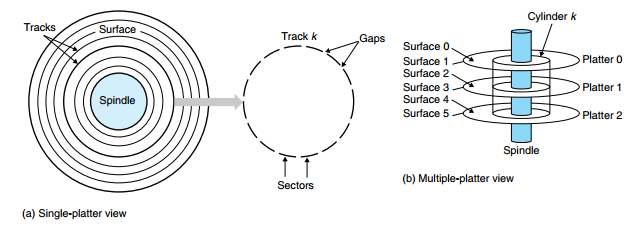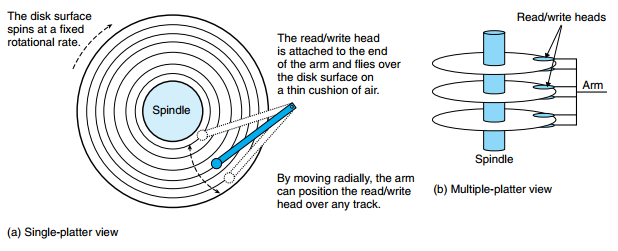# 磁盘

## 1. 构造

• 盘片（platter）：每个盘片有两面，表面覆盖着磁性记录材料。
• 主轴（spindle）：使得盘片易固定的旋转速率旋转。
• 磁道（track）：每个磁盘表面有一组同心圆磁道构成。
• 扇区（sector）：每个磁道被划分为一组扇区，每个扇区包含相等数量的数据位（通常位 512 字节）
• 间隙（gap）：扇区之间由间隙分隔开。间隙中不存储数据位，用于标识扇区的格式化位。
• 柱面（cylinder）：所有盘片表面上到主轴中心的距离相等的磁道的集合。## 2. 容量

• 记录密度（recording density）（单位：位/英寸）：磁盘一英寸的段中可以放入的位数。

• 磁道密度（track density）（道/英寸）：从盘片中心出发半径上一英寸的段内可以有的磁道数。

• 面密度（areal density）（位/平方英寸）：记录密度与磁道密度的乘积。

$\begin{array}{c} \text{磁盘容量} = {\text{字节数} \over \text{扇区}} \times {\text{平均扇区数} \over \text{磁道}} \times {\text{磁道数} \over \text{表面}} \times {\text{表面数} \over \text{盘片}} \times {\text{盘片数} \over \text{磁盘}} \\ = \text{单位扇区的字节数} \times \text{单位磁道的平均扇区数} \times \text{单位表面的磁道数} \\ \times \text{单位盘片的表面数} \times \text{单位磁盘的盘片数} \end{array}$

#### 【注意】

• 对于 DRAM 和 SRAM 容量相关的计量单位，通常 $K = 2^{10}, M = 2^{20}, G = 2^{30}, T = 2^{40}$
• 对于像磁盘和 I/O 设备容量相关的计算单位，通常 $K = 10^{3}, M = 10^{6}, G = 10^{9}, T = 10^{12}$

## 3. 操作• 寻道时间（seek time）：为了读取某个目标扇区的内容，传动臂将读/写头定位到包含目标扇区的磁道上所有的时间称为寻道时间。现代驱动器中平均寻道时间 $T_{avg seek}$ 是通过对几千次对随机扇区的寻道平均值来测量的，通常为 $3 \sim 9$ ms。

• 旋转时间（rotational latency）：读/写头到了期望的磁道后，驱动器等待目标扇区的第一个位旋转到读/写头下的时间。最大旋转时间是读/写头达到期望的磁道时刚好错过了目标扇区，因此必须等待磁盘旋转一整圈，故最大旋转时间为

$\begin{array}{c} T_{max \ rotation} = {1 \over RPM} \times {60 \ s\over 1 \ min} \end{array}$

$\begin{array}{c} T_{avg \ rotation} = {1 \over 2}T_{max \ rotation} \end{array}$

• 传送时间（transfer time）：当目标扇区的第一个位位于读/写头下时，驱动器开始读/写该扇区的内容，读/写过程中数据的传输时间称为传送时间。

$\begin{array}{c} T_{avg \ transfer} = {1 \over RPM} \times {1 \over \text{(平均扇区数 / 磁盘)}} \times {60 \ s \over 1 \ min} \end{array}$

## 4. 逻辑磁盘块

• 磁盘封装中有一个小的硬盘/固件设备，称为磁盘控制器，维护着逻辑块号和实际（物理）磁盘扇区之间的映射关系。

• 当操作系统想要执行一个 I/O 操作时，比如读取一个磁盘扇区的数据到主存，操作系统会发送一个命令到磁盘控制器，让它读某个逻辑块号。

• 控制器上的固件执行一个快速表查找，将一个逻辑块号翻译成一个（盘面，磁道，扇区）的三元组，这个三元组唯一地标识了对应的物理扇区。

• 控制器上的硬件会解释这个三元组，将读/写头移动到适当的柱面，等待扇区转动到读/写头下，将读/写头感知到的位放到控制器上的一个小缓冲区中，然后将它们复制到主存中。

#### 格式化的磁盘容量

• 用标识扇区的信息填写扇区之间的间隙
• 标识出有故障的柱面并且不使用它们
• 在每个区中预留出一组柱面作为备用，当其区中某个柱面坏掉了可以替换使用（因此磁盘制造商所说的格式化容量比最大容量要小）